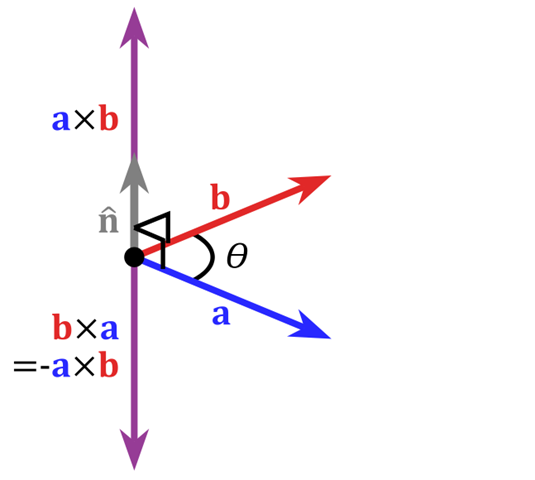机器学习中向量的点积和叉乘含义梳理

• 时间:
• 浏览:

定义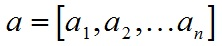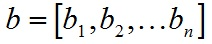a和b的点积公式为：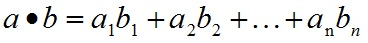向量内积的性质：

1. a^2 ≥ 0；当a^2 = 0时，必有a = 0. （正定性）
2. a·b = b·a. （对称性）
3. a + μbc = λa·c + μb·c，对任意实数λ, μ成立. （线性）
4. cos∠(a,b) =a·b/(|a||b|).
5. |a·b| ≤ |a||b|，等号只在ab共线时成立.

向量内积的几何意义

1. 表征或计算两个向量之间的夹角
2. b向量在a向量方向上的投影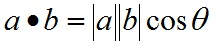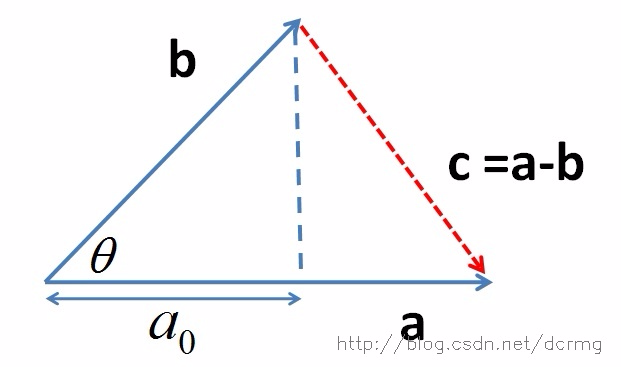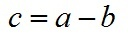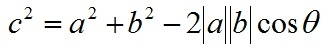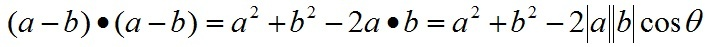a∙b=|a||b|cos⁡(θ)

θ=arccos⁡(a∙b|a||b|)

a∙b>0→方向基本相同，夹角在0°到90°之间
a∙b=0→ 正交，相互垂直
a∙b<0→ 方向基本相反，夹角在90°到180°之间

向量的外积（叉乘）

定义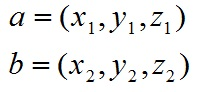a和b的外积公式为：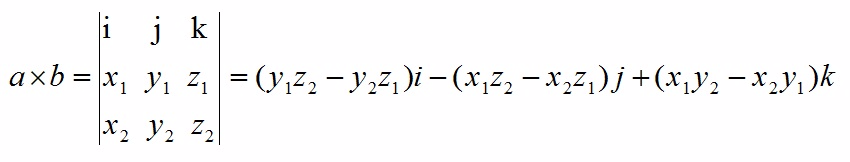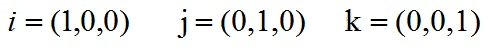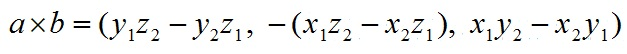向量外积的性质

1. a × b = -b × a. （反称性）
2. a + μb) × c = λ(a ×c) + μ(b ×c). （线性）

向量外积的几何意义